# Pilsen Region

Between 2000 and 2001, 14 per mille of the population decreased in the Pilsen Region. In 2000, the Pilsen Region had 551281 inhabitants. If the declining trend continues the same (i. E. , 14 per mille of inhabitants per year), how many inhabitants will the Pilsen Region have in 2100?

n =  136517

### Step-by-step explanation:Did you find an error or inaccuracy? Feel free to write us. Thank you!Tips to related online calculators
Do you want to round the number?
Do you want to convert time units like minutes to seconds?

## Related math problems and questions:

• Pilsen circus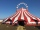The arrival of the circus in Pilsen was seen in the morning at 08:00 by a city citizen. He passed this information on 08:15 to three other residents of the city. Each of these three people then informed the other three residents at 08:30, and again at 08:
• DemographicsThe population grew in the city in 10 years from 30000 to 34000. What is the average annual percentage increase of population?
• Annual growthThe population has grown from 25,000 to 33,600 in 10 years. Calculate what was the average annual population growth in%?
• PopulationThe town has 65,000 inhabitants. 40 years ago, there were 157,000. How many people will live in a city in 10 years if the population's average rate is as in previous years?
• The town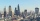The town population is 56000. It is decreasing by 2% every year. What will be the population of the town after 13 years?
• The city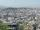At the end of 2010 the city had 248000 residents. The population increased by 2.5% each year. What is the population at the end of 2013?
• Geometric progressionIn geometric progression, a1 = 7, q = 5. Find the condition for n to sum first n members is: sn≤217.
• Volume of woodEvery year, at the same time, an increase in the volume of wood in the forest is measured. The increase is regularly p% compared to the previous year. If in 10 years the volume of wood has increased by 10%, what is the number p?
• Q of GP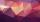Calculate quotient of geometric progression if a1=5 a a1+a2=12.
• Population growth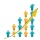How many people will be on Earth from two people for 5,000 years, if every couple has always 4 children, (2 boys and 2 girls) at the age of 25-35, and every man will live 75 years?
• PopulationWhat is the population of the city with 3% annual growth, if in 10 years the city will have 60,000 residents?
• Geometric progressiobIf the sum of four consective terms of geometric progression is 80 and arithmetic mean of second and fourth term is 30 then find terms?
• Growth of woodThe annual growth of wood in the forest is estimated at 2%. In how many years will make the forest volume double?
• Quotient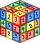Determine the quotient and the second member of the geometric progression where a3=10, a1+a2=-1,6 a1-a2=2,4.
• Fish population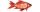The number of fish in a lake decreased by 10% during period of one year. Last year there were 500 fish in the lake. What is the fish population this year?
• Microorganisms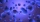The first generation of micro-organisms has a population of 13500 members. Each next generation is 11/10 times the previous one. Find out how many generations will reach at least three times members of the first generation.
• LoanApply for a \$ 59000 loan, the loan repayment period is 8 years, the interest rate 7%. How much should I pay for every month (or every year if paid yearly). Example is for practise geometric progression and/or periodic payment for an annuity.